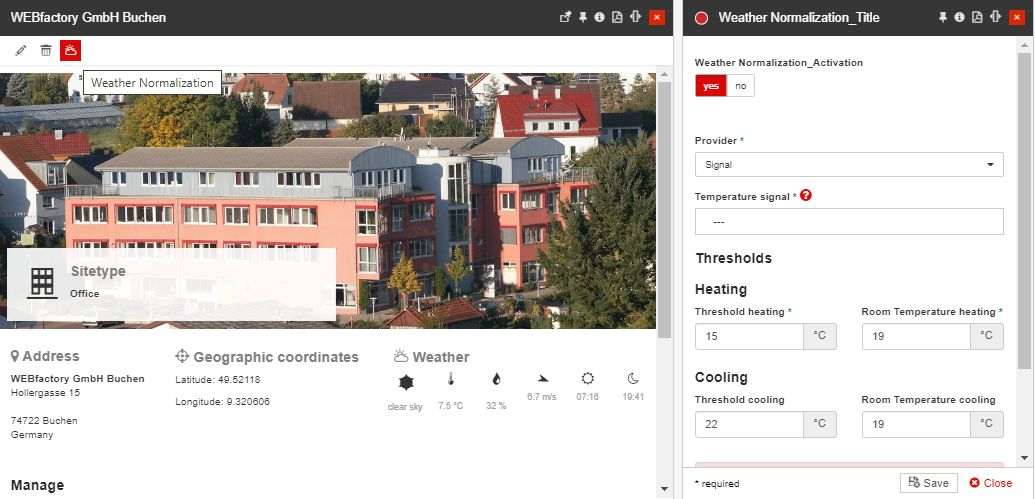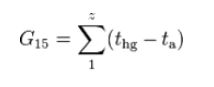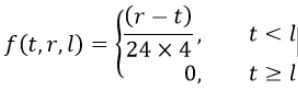# i4connected Knowledgebase 5.6

### The Weather Normalization mechanism

Abstract

Weather Normalization measures the impact of weather on energy consumption. Because weather patterns vary widely day-to-day and year-to-year, weather for a given season may be colder or warmer. The used energy is directly dependent on how cold it is. Comparing the weather or energy consumption from one year to the next would provide only the change between those years. However, when energy consumption is “Weather Normalized” you are comparing your energy consumption over a normal weather period. Weather normalization adjusts energy usage so it can be compared to the energy used in other years over a longer period of time.

The Weather Normalization panel can be accessed from the toolbar of the Site detailed view mode, by clicking the Weather Normalization button.The Weather Normalization button, in the Site context

As the Weather Normalisation has been implemented based on the VDI 3807 norm, the calculation formula is:The calculation formula

where:

• z = number of measured heating days of the heating season, based on the individual heating limit;

• thg = heating limit;

• ta= average outdoor temperature of each heating day.

If the temperature is collected in 15 minute periods or interpolated to such interval, the Heating Degree Days (HDD) should be calculated directly by the function:Function

where:

• t = measured air temperature;

• r = room temperature;

• l = temperature limit.

#### Public weather data

The public weather data should be collected from a certified weather service using a specific adapter. The weather signals should be organized in a way easy for the user to select when setting up the weather normalization. Only the temperature signal should be collected it should be stored as an adapter signal.

The Degree Days calculation should be done via a virtual signal that is linked only to the source temperature signal chosen for each site. Each site should have its Degree Days signals and each signal should be part of a Degree Days Signal Type/KPI.

Implementation

Calculation

IIf(IsLeaf([Site].[Site].CurrentMember),
([Measures].[Sum], [Signal].[Type].&) * DIVIDE(Avg(
{ ParallelPeriod ([Time].[Calendar].[Year], 01, [Time].[Calendar].CurrentMember),
ParallelPeriod ([Time].[Calendar].[Year], 02, [Time].[Calendar].CurrentMember),
ParallelPeriod ([Time].[Calendar].[Year], 03, [Time].[Calendar].CurrentMember),
ParallelPeriod ([Time].[Calendar].[Year], 04, [Time].[Calendar].CurrentMember),
ParallelPeriod ([Time].[Calendar].[Year], 05, [Time].[Calendar].CurrentMember),
ParallelPeriod ([Time].[Calendar].[Year], 06, [Time].[Calendar].CurrentMember),
ParallelPeriod ([Time].[Calendar].[Year], 07, [Time].[Calendar].CurrentMember),
ParallelPeriod ([Time].[Calendar].[Year], 08, [Time].[Calendar].CurrentMember),
ParallelPeriod ([Time].[Calendar].[Year], 09, [Time].[Calendar].CurrentMember),
ParallelPeriod ([Time].[Calendar].[Year], 10, [Time].[Calendar].CurrentMember)} * [Signal].[Type].&,
[Measures].[Sum]), ([Measures].[Sum], [Signal].[Type].&)),
RollUpChildren([Site].[Site].CurrentMember,'+'))

Unit

kWh

Category

Electricity

Sources

• Building 1 (Site)

• Energy Meter 1 (Device)

• Energy (kWh) (Signal)

• Water (m³) (Signal)

• Weather Station (Device

• Temperature (ºC) (Signal) this could either be a real signal or a virtual signal copying the value from another weather station.

• Heating Degree Days (HDD) (Virtual Signal) = Temperature < 15 ? (20 - Temperature) / 24 / 4 : 0;

• Cooling Degree Days (CDD) (Virtual Signal) = Temperature > 25 ? (Temperature - 20) / 24 / 4 : 0;

Sample Query:

WITH 	MEMBER [Consumption] AS ([Measures].[Sum], [Signal].[Type].[Heating])
MEMBER [HDD] AS ([Measures].[Sum], [Signal].[Type].[Heating Degree Days])
MEMBER [Avg HDD] AS Avg(
{ ParallelPeriod ([Time].[Calendar].[Year], 01, [Time].[Calendar].CurrentMember),
ParallelPeriod ([Time].[Calendar].[Year], 02, [Time].[Calendar].CurrentMember),
ParallelPeriod ([Time].[Calendar].[Year], 03, [Time].[Calendar].CurrentMember),
ParallelPeriod ([Time].[Calendar].[Year], 04, [Time].[Calendar].CurrentMember),
ParallelPeriod ([Time].[Calendar].[Year], 05, [Time].[Calendar].CurrentMember),
ParallelPeriod ([Time].[Calendar].[Year], 06, [Time].[Calendar].CurrentMember),
ParallelPeriod ([Time].[Calendar].[Year], 07, [Time].[Calendar].CurrentMember),
ParallelPeriod ([Time].[Calendar].[Year], 08, [Time].[Calendar].CurrentMember),
ParallelPeriod ([Time].[Calendar].[Year], 09, [Time].[Calendar].CurrentMember),
ParallelPeriod ([Time].[Calendar].[Year], 10, [Time].[Calendar].CurrentMember)} * [Signal].[Type].[Heating Degree Days],
[Measures].[Sum])
MEMBER [Ratio] AS DIVIDE([Avg HDD], [HDD])
MEMBER [Normalized] AS IIf(IsLeaf([Site].[Site].CurrentMember),
[Consumption] * [Ratio],
RollUpChildren([Site].[Site].CurrentMember,'+'))
SELECT NON EMPTY { [Normalized] } ON COLUMNS,
NON EMPTY [Time].[Month].[Month] ON ROWS
FROM (
SELECT [Time Zone].[Time Zone].& ON COLUMNS
FROM (
SELECT [Time].[Year].&[2012-01-01T00:00:00] ON COLUMNS
FROM [WEBfactory DWH]
)
)